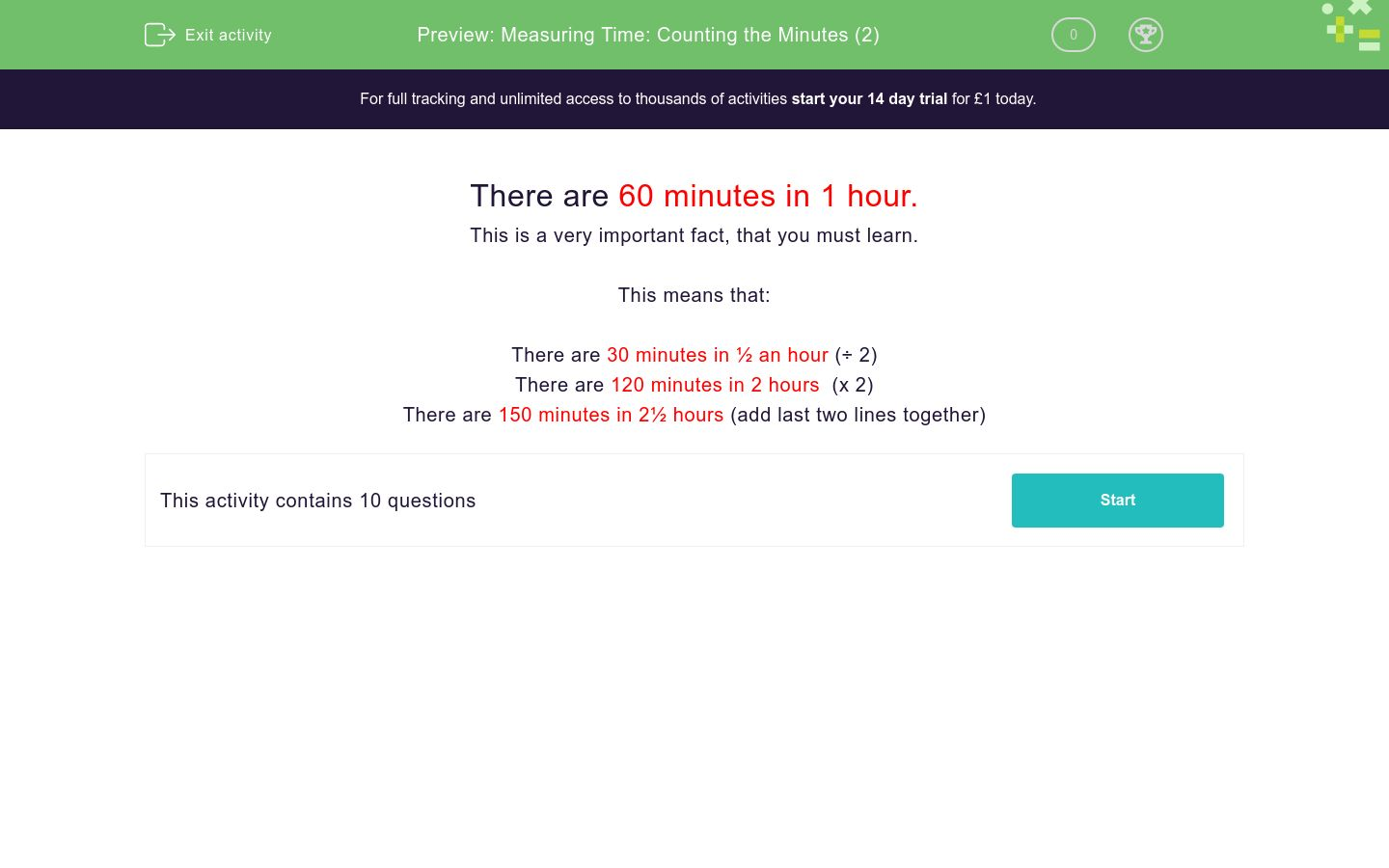# Measuring Time: Counting the Minutes (2)

In this worksheet, children must convert between hours and minutes.Key stage:  KS 1

Curriculum topic:   Measurement

Curriculum subtopic:   Know Divisions of Time

Difficulty level:### QUESTION 1 of 10

There are 60 minutes in 1 hour.

This is a very important fact, that you must learn.

This means that:

There are 30 minutes in ½ an hour (÷ 2)

There are 120 minutes in 2 hours (x 2)

There are 150 minutes in 2½ hours (add last two lines together)

How many minutes are there in ½ hour?

(just write the number)

How many minutes are there in 2 hours?

(just write the number)

How many minutes are there in 2½ hours?

(just write the number)

How many minutes are there in 1½ hours?

(just write the number)

How many minutes are there in 3 hours?

(just write the number)

How many hours are there in 120 minutes?

(just write the number)

How many hours are there in 180 minutes?

(just write the number)

How many hours are there in 240 minutes?

(just write the number)

How many hours are there in 90 minutes?

2

How many hours are there in 150 minutes?

2

• Question 1

How many minutes are there in ½ hour?

(just write the number)

30
• Question 2

How many minutes are there in 2 hours?

(just write the number)

120
• Question 3

How many minutes are there in 2½ hours?

(just write the number)

150
• Question 4

How many minutes are there in 1½ hours?

(just write the number)

90
• Question 5

How many minutes are there in 3 hours?

(just write the number)

180
• Question 6

How many hours are there in 120 minutes?

(just write the number)

2
• Question 7

How many hours are there in 180 minutes?

(just write the number)

3
• Question 8

How many hours are there in 240 minutes?

(just write the number)

4
• Question 9

How many hours are there in 90 minutes?

• Question 10

How many hours are there in 150 minutes?